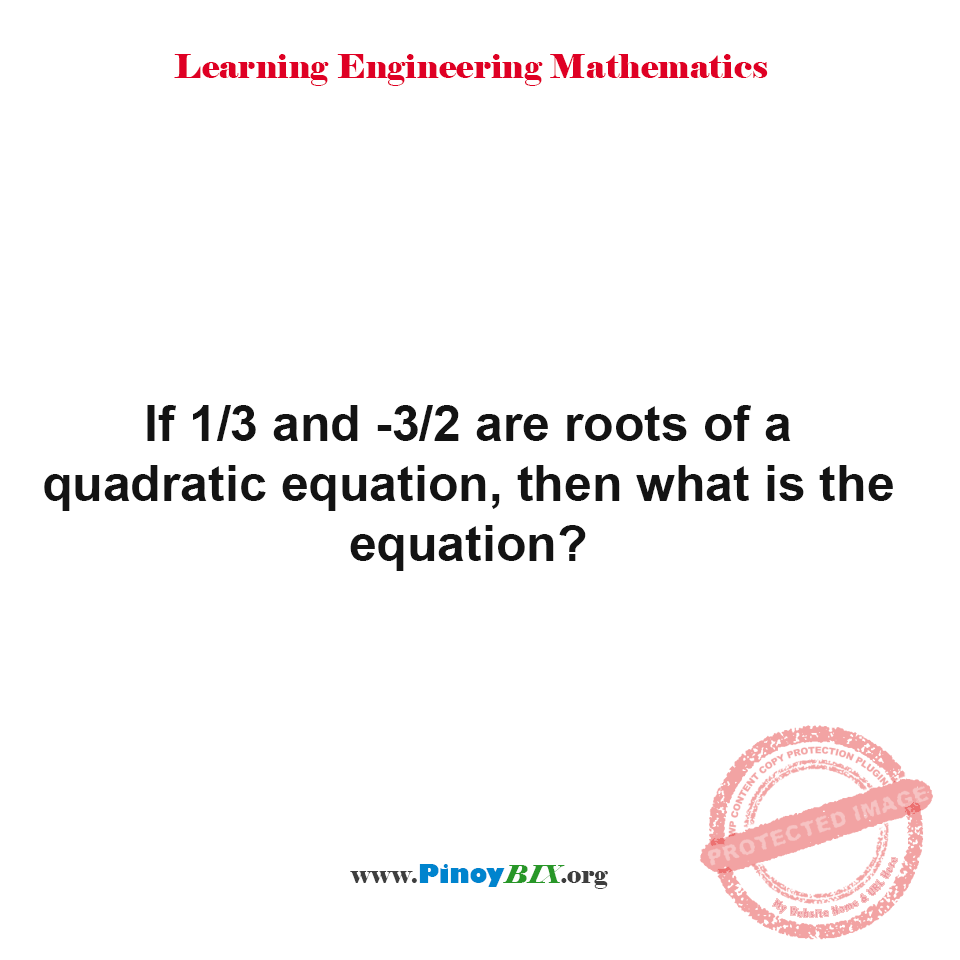# Solution: If 1/3 and -3/2 are roots of a quadratic equation, then what is the equation?

(Last Updated On: January 21, 2020)#### Problem Statement:

If 1/3 and -3/2 are roots of a quadratic equation, then what is the equation?

• A. 6x^2 + 7x – 3 = 0
• B. 6x^2 – 7x + 3 = 0
• C. 6x^2 – 7x – 3 = 0
• D. 6x^2 – 7x + 1 = 0

The equation is equal to 6x^2 + 7x – 3 = 0.

#### Latest Problem Solving in Quadratic Equation, Binomial Theorem and Logarithms

More Questions in: Quadratic Equation, Binomial Theorem and Logarithms

#### Online Questions and Answers in Quadratic Equation, Binomial Theorem and Logarithms

Show Simple Act of Caring!

Please consider subscribing to our YouTube Channel for engineering video tutorials. You can also find us on Twitter and Facebook. Lastly, Our app is now available on Google Play, Pinoybix Elex

© 2014 PinoyBIX Engineering. © 2019 All Rights Reserved | How to Donate? |#### Pre-board in Electronics Engineering

Pre-board in Electronics Engineering - Answers

#### Pre-board in Communications Engineering

Pre-board in Communications Engineering - Answers

#### GEAS Solution

Dynamics problem Economics problem Physics problem Statics problem Strength problem Thermodynamics problem

#### Questions and Answers in GEAS

Engineering Economics Engineering Laws and Ethics Engineering Management Engineering Materials Engineering Mechanics General Chemistry Physics Strength of Materials Thermodynamics
Consider Simple Act of Caring!: LIKE MY FB PAGE

Our app is now available on Google Play, Pinoybix Elex